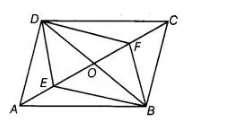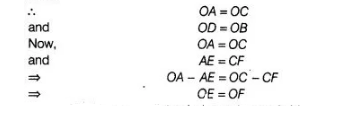# E and F are points on diagonal`
Question:

E and F are points on diagonal AC of a parallelogram ABCD such that AE = CF. Show that BFDE is a parallelogram.

Solution:

Given $A B C D$ is a parallelogram and $A E=C F$

To show $O E=O F$

Construction Join $B D$, meet $A C$ at point $O$.

Proof Since, diagonals of a parallelogram bisect each other.Thus, BFDE is a quadrilateral whose diagonals bisect each other. Hence, $B F D E$ is a parallelogram.

Hence proved.